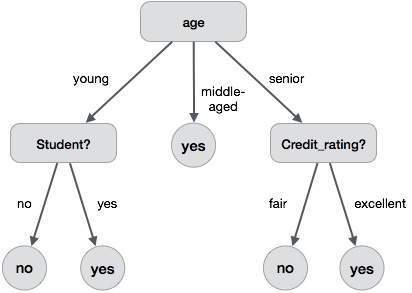# Data Mining - Decision Tree Induction

A decision tree is a structure that includes a root node, branches, and leaf nodes. Each internal node denotes a test on an attribute, each branch denotes the outcome of a test, and each leaf node holds a class label. The topmost node in the tree is the root node.

The following decision tree is for the concept buy_computer that indicates whether a customer at a company is likely to buy a computer or not. Each internal node represents a test on an attribute. Each leaf node represents a class.The benefits of having a decision tree are as follows −

• It does not require any domain knowledge.
• It is easy to comprehend.
• The learning and classification steps of a decision tree are simple and fast.

## Decision Tree Induction Algorithm

A machine researcher named J. Ross Quinlan in 1980 developed a decision tree algorithm known as ID3 (Iterative Dichotomiser). Later, he presented C4.5, which was the successor of ID3. ID3 and C4.5 adopt a greedy approach. In this algorithm, there is no backtracking; the trees are constructed in a top-down recursive divide-and-conquer manner.

```Generating a decision tree form training tuples of data partition D
Algorithm : Generate_decision_tree

Input:
Data partition, D, which is a set of training tuples
and their associated class labels.
attribute_list, the set of candidate attributes.
Attribute selection method, a procedure to determine the
splitting criterion that best partitions that the data
tuples into individual classes. This criterion includes a
splitting_attribute and either a splitting point or splitting subset.

Output:
A Decision Tree

Method
create a node N;

if tuples in D are all of the same class, C then
return N as leaf node labeled with class C;

if attribute_list is empty then
return N as leaf node with labeled
with majority class in D;|| majority voting

apply attribute_selection_method(D, attribute_list)
to find the best splitting_criterion;
label node N with splitting_criterion;

if splitting_attribute is discrete-valued and
multiway splits allowed then  // no restricted to binary trees

attribute_list = splitting attribute; // remove splitting attribute
for each outcome j of splitting criterion

// partition the tuples and grow subtrees for each partition
let Dj be the set of data tuples in D satisfying outcome j; // a partition

if Dj is empty then
attach a leaf labeled with the majority
class in D to node N;
else
attach the node returned by Generate
decision tree(Dj, attribute list) to node N;
end for
return N;
```

## Tree Pruning

Tree pruning is performed in order to remove anomalies in the training data due to noise or outliers. The pruned trees are smaller and less complex.

### Tree Pruning Approaches

There are two approaches to prune a tree −

• Pre-pruning − The tree is pruned by halting its construction early.

• Post-pruning - This approach removes a sub-tree from a fully grown tree.

## Cost Complexity

The cost complexity is measured by the following two parameters −

• Number of leaves in the tree, and
• Error rate of the tree.